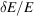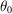International Tables for Crystallography (2006). Vol. C, ch. 2.5, pp. 84-88https://doi.org/10.1107/97809553602060000580

## Contents

• 2.5. Energy-dispersive techniques  (pp. 84-88)
• 2.5.1. Techniques for X-rays  (pp. 84-87) | html | pdf |
• 2.5.1.1. Recording of powder diffraction spectra  (p. 84) | html | pdf |
• 2.5.1.2. Incident X-ray beam  (p. 84) | html | pdf |
• 2.5.1.3. Resolution  (p. 85) | html | pdf |
• 2.5.1.4. Integrated intensity for powder sample  (pp. 85-86) | html | pdf |
• 2.5.1.5. Corrections  (p. 86) | html | pdf |
• 2.5.1.6. The Rietveld method  (p. 86) | html | pdf |
• 2.5.1.7. Single-crystal diffraction  (p. 86) | html | pdf |
• 2.5.1.8. Applications  (pp. 86-87) | html | pdf |
• 2.5.2. White-beam and time-of-flight neutron diffraction  (pp. 87-88) | html | pdf |
• 2.5.2.1. Neutron single-crystal Laue diffraction  (p. 87) | html | pdf |
• 2.5.2.2. Neutron time-of-flight powder diffraction  (pp. 87-88) | html | pdf |
• References | html | pdf |
• Figures
• Fig. 2.5.1.1. Standard and conical diffraction geometries: 2θ 0 = fixed scattering angle  (p. 84) | html | pdf |
• Fig. 2.5.1.2. XED powder spectrum of BaTiO 3 recorded with synchrotron radiation from the electron storage ring DORIS at DESY-HASYLAB in Hamburg, Germany  (p. 84) | html | pdf |
• Fig. 2.5.1.3. Relative resolution,, as function of Bragg angle,, for two values of the lattice plane spacing: ( a ) 1 Å and ( b ) 0.5 Å  (p. 85) | html | pdf |
• Fig. 2.5.2.1. Construction in reciprocal space to illustrate the use of multi-wavelength radiation in single-crystal diffraction  (p. 87) | html | pdf |